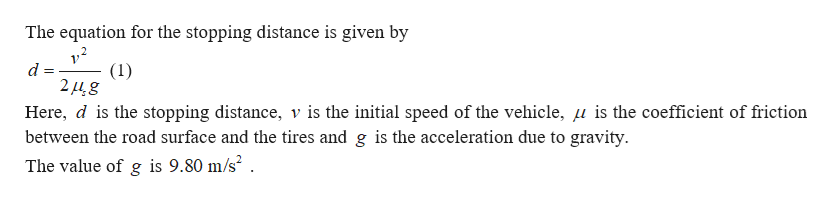# A car is traveling at 50.0 mi/h on a horizontal highway.a) If the coefficient of static friction between road and tires on a rainy day is 0.100, what is the minimum distance in which the car will stop? (in m)b) What is the stopping distance when the surface is dry and µs = 0.602? (in m)

Question
219 views

A car is traveling at 50.0 mi/h on a horizontal highway.

a) If the coefficient of static friction between road and tires on a rainy day is 0.100, what is the minimum distance in which the car will stop? (in m)

b) What is the stopping distance when the surface is dry and µs = 0.602? (in m)

check_circle

Step 1help_outlineImage TranscriptioncloseThe equation for the stopping distance is given by 1/ d = (1) 2448 Here, d is the stopping distance, v is the initial speed of the vehicle, u is the coefficient of friction between the road surface and the tires and g is the acceleration due to gravity The value of g is 9.80 m/s2 . fullscreen

### Want to see the full answer?

See Solution

#### Want to see this answer and more?

Solutions are written by subject experts who are available 24/7. Questions are typically answered within 1 hour.*

See Solution
*Response times may vary by subject and question.
Tagged in

### Physics